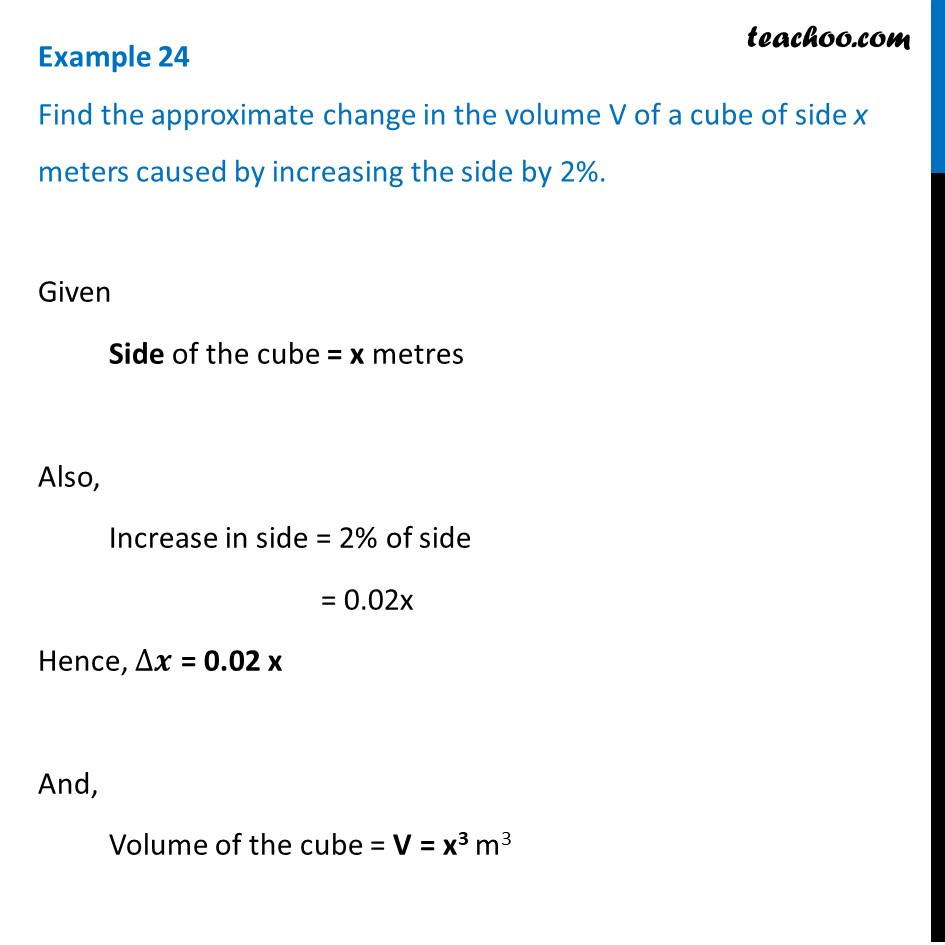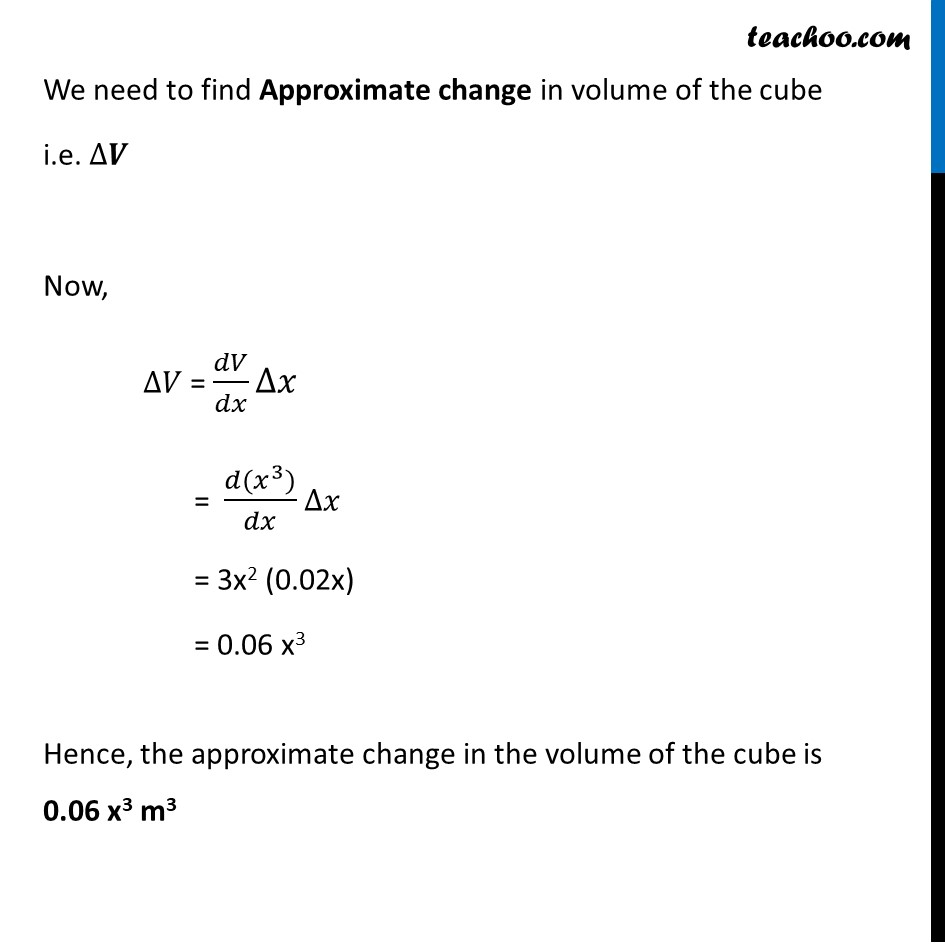Finding approximate value- Statement questions

Chapter 6 Class 12 Application of Derivatives (Term 1)
Concept wise### Transcript

Example 24 Find the approximate change in the volume V of a cube of side x meters caused by increasing the side by 2%.Given Side of the cube = x metres Also, Increase in side = 2% of side = 0.02x Hence, ∆𝒙 = 0.02 x And, Volume of the cube = V = x3 m3 We need to find Approximate change in volume of the cube i.e. ∆𝑽 Now, ∆𝑉 = 𝑑𝑉/𝑑𝑥 ∆𝑥" " = (𝑑(𝑥^3))/𝑑𝑥 ∆𝑥" " = 3x2 (0.02x) = 0.06 x3 Hence, the approximate change in the volume of the cube is 0.06 x3 m3